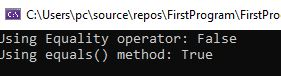﻿ C# == vs equal() method - TutorialsTrend
This Angular tutorial helps you get started with Angular quickly and effectively through many practical examples.
`

# C# - == vs equal() Method

Many developers get into situations while writing code when they must use the equal() method or the == operator. We shall examine how they vary practically in this tutorial. In C#, the == operator and the Equal() method are used for comparing the equality of two objects or values, but they differ in their behavior and usage.

#### The == operator

The == operator can be overloaded for any type, not just string. It is used to compare the equality of two objects or values in C#. It can be used to compare value types, reference types, and nullable value types. When comparing value types, the == operator compares their values. When comparing reference types, the == operator compares whether both objects refer to the same memory location. If two reference types have the same values but different memory locations, the == operator will return false.

The Equal() method

It is a method defined in the Object class and is used to compare the equality of two objects. It is often used to compare strings and other reference types in C#. The Equal() method compares the values of the objects, not their memory locations. When comparing strings, it is generally recommended to use the Equal() method instead of the == operator because the Equal() method is more reliable when comparing strings.

#### Example1

Here is an example of using == operator and Equal() method to compare two strings.

string str1 = "Rohatash";
string str2 = "Rohatash";
bool isEqual = str1 == str2; // Returns true
bool isEqualMethod = str1.Equal(str2); // Returns true

In this example, both the == operator and the Equal() method return true because the values of the two strings are equal.

Example2 (Main Difference)

If you use the == operator to compare two reference types with the same values but different memory locations, it will return false. This is because the == operator compares the memory locations of the two objects, not their values. Even if two objects have the same values, they may be located in different memory locations, which makes them different objects in terms of reference types.

Example

``````using System;
using System.Text;

namespace FirstProgram
{
class Program
{
static void Main(string[] args)
{
object str = "Rohatash";
char[] values = { 'R', 'o', 'h', 'a', 't','a','s','h' };
object str2 = new string(values);
Console.WriteLine("Using Equality(==) operator: {0}", str == str2);
Console.WriteLine("Using equals() method: {0}", str.Equals(str2));